A quantitative analysis of XKCD comic #314

In this post, we're going to analyze an XKCD comic about dating pools and derive the statistical analysis that's behind the curves shown in the comic.

First of all, let's take a look at the comic:

In :
from IPython.display import Image
Image(url="http://imgs.xkcd.com/comics/dating_pools.png")
Out: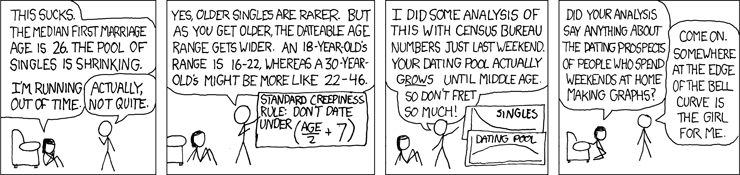As you can see, the comic mentions some analysis involving census bureau data and dating pools. Our goal in this post is to replicate the analysis that leads to these curves. We proceed in two parts:

• first, we will take a closer look at the "standard creepiness rule"
• then, we'll apply the creepiness rule to census bureau data available on the internet and see if we get the same type of plot shown in the comic

The "standard creepiness rule"¶

As explained in the comic, the "standard creepiness rule" implies that you can't date people below a certain age that depends on your own age. Assuming this applies to everyone in the population or society you live in, this means that there also is an upper age bound over which people can't date you because you're too young for them.

Formally, we can denote these two bounds as:

• youngest_person_you_can_date = creepiness_rule(your_age)
• your_age = creepiness_rule(oldest_person_that_can_date_you)

Still more mathematically, if we denote the creepiness rule by $f$, upper and lower bounds by $\text{upper_bound}$ and $\text{lower_bound}$ and your age by $x$ then we have:

$$\text{lower_bound} = f(x)$$$$f(\text{upper_bound}) = x \Leftrightarrow \text{upper_bound} = f^{-1}(x)$$

This can be easily implemented, as the inverse creepiness rule is "you can't date people older than twice (your age minus 7 years)".

In :
def creepiness_rule(age):
return age / 2. + 7

def inverse_creepiness_rule(age):
return 2. * (age - 7)

We can prepare a little plot to look at the lower and upper bounds as a function of your age:

In :
from pylab import *
%matplotlib inline
In :
ages = linspace(15, 70)
plot(ages, creepiness_rule(ages), label='youngest people you can date')
plot(ages, inverse_creepiness_rule(ages), label='oldest people you can date')
legend(loc=2)
xlim((15, 70))
grid(True)
ylabel("age (years)")
Out: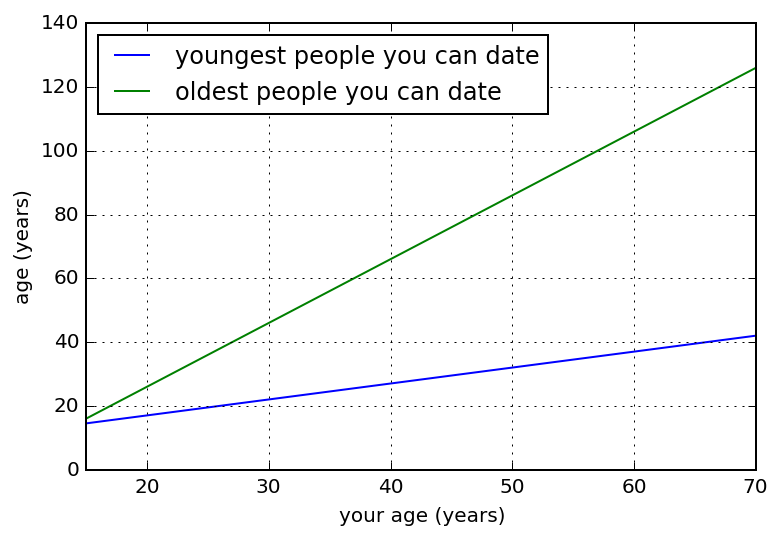As one can see from the plot above, the dating interval is getting larger with your age. When computed, the interval can be found to be equal to $\frac{3}{2}age - 14$.

Therefore, what I would call "the dating pool paradox" results from two factors that have inverse effects on the dating pool:

• your dating age interval grows by a factor 1.5
• while at the same time the number of single decreases

We will explore this phenomenon with some real data in the next section. But first, we'll need to define a dating bounds function and check that it returns the numbers given in the comic.

In :
def dating_bounds(age):
return (creepiness_rule(age), inverse_creepiness_rule(age))

When you're 18, you can date people in the following interval:

In :
dating_bounds(18)
Out:
(16.0, 22.0)

When you're 30, your dating pool is in the following age group:

In :
dating_bounds(30)
Out:
(22.0, 46.0)

In the next section, we apply the previous functions to some real numbers to see if we can indeed derive the dating pool curves Randall Munroe is showing in his comic.

Including census bureau numbers into the analysis¶

Getting the data¶

Figures such as the ones said to exist in the comic can indeed be found on the interwebz: http://www.census.gov/population/www/socdemo/hh-fam/cps2011.html. Downloading the excel file at http://www.census.gov/population/socdemo/hh-fam/cps2011/tabA1-all.xls, we can continue the analysis and replicate Randall's findings. I edited the data found in the csv file to compute the age pools of singles by considering that the "single person" category is the union of the categories "Married Spouse Absent", "Widowed", "Divorced", "Separated" and "Never Married".

The data is given as the number of singles within an age category bounded by lower and upper ages. As an example, there are 7993 thousand singles of both sexes between the ages of 18 and 19.

In :
ages_lower = array([15, 18, 20, 25, 30, 35, 40, 45, 50, 55, 65, 75, 85])
ages_upper = array([17, 19, 24, 29, 34, 39, 44, 49, 54, 64, 74, 84, 100])
data_both_sexes = array([12740, 7993, 19063, 13970, 9233, 7277, 7403, 7971, 7708, 12733, 7825, 6206, 3428])
data_males = array([6559, 4082, 10079, 7726, 4952, 3639, 3729, 3860, 3557, 5461, 2614, 1592, 826])
data_females = array([6183, 3910, 8986, 6244, 4281, 3638, 3673, 4112, 4151, 7272, 5212, 4616, 2601])

Due to the irregular sampling of the data, the next section will be devoted to building an interpolation function that returns the number of singles between given age bounds.

Building an interpolation function on the data¶

In this section, we'll be writing a function that computes the number of singles between two age bounds. We first compute the number of singles above a certain age:

In :
def singles_above_age(age, data):
"""
returns the number of singles found in vector data above a given age
"""
if age >= ages_lower[-1]:
return float(ages_upper[-1] - age) / (ages_upper[-1] - ages_lower[-1] + 1) * data[-1]
ind = (ages_lower >= age).nonzero()
singles = sum(data[ind:])
if ind != 0: #
delta_age = ages_lower[ind] - age
singles += float(delta_age) / (ages_upper[ind - 1] - ages_lower[ind - 1] + 1) * data[ind - 1]
return singles

Let's check if the function works.

In :
print(singles_above_age(20, data_females))
print(sum(data_females[2:]))
54786.0
54786
In :
print(singles_above_age(19, data_females))
print(sum(data_females[2:]) + data_females / 2)
56741.0
56741.0
In :
print(singles_above_age(18.5, data_females))
print(sum(data_females[2:]) + data_females / 2 * 1.5)
57718.5
57718.5
In :
print(singles_above_age(99, data_females))
print(data_females[-1] / 16)
162.5625
162.5625

This seems to work. So let's build a function that returns singles between two age bounds. We are using the fact that the number of people between age1 and age2 are all those above age1 minus the ones above age2.

In :
def singles_between_bounds(bounds, data):
return singles_above_age(bounds, data) - singles_above_age(bounds, data)

Let's check that the function works.

In :
print(singles_between_bounds((15, 18), data_males))
print(data_males)
6559.0
6559

This is quite neat. We can now move on to the analysis we want to conduct.

Plotting singles as a function of age (correctly sampled, this time)¶

First, let's look at the number of singles in 1 year intervals between 18 and 80!

In :
ages = arange(18, 80, 1)
both = [singles_between_bounds((age, age + 1), data_both_sexes) for age in ages]
males = [singles_between_bounds((age, age + 1), data_males) for age in ages]
females = [singles_between_bounds((age, age + 1), data_females) for age in ages]
plot(ages, both, label="both sexes")
plot(ages, males, label="males")
plot(ages, females, label="females")
xlabel("age class (lower bound)")
ylabel("singles (in thousands)")
title("number of singles as a function of age class")
legend()
grid()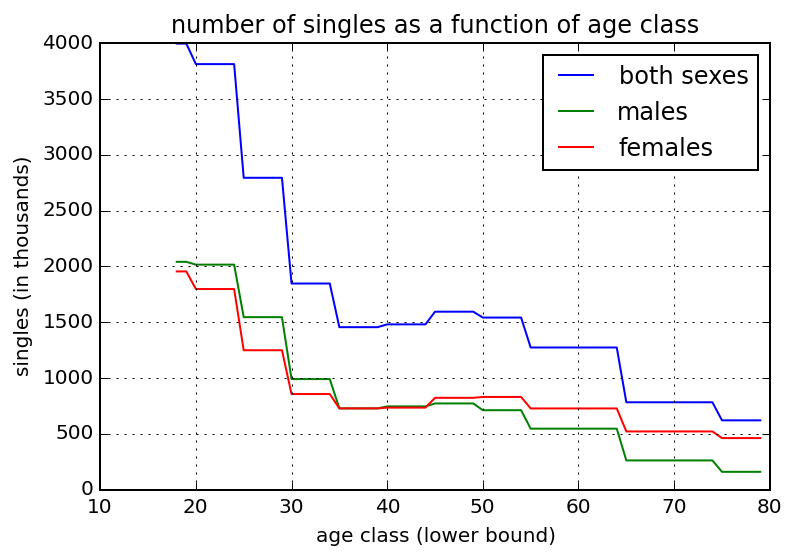It's interesting to comment on this curve:

• the number of singles is strongly declining between ages 20 and 30
• there is a rebound around the mid-40's: is this the mid-life crisis?
• after the rebound, the number of singles diminishes again
• trends for men and women are quite similar except for the fact that the mid-40's rebound reaches a higher peak for women

Now that our preliminary work is done, we can easily compute dating pools using the previously defined functions.

Plotting the dating pool using the standard creepiness function defined in the XKCD comic¶

We define a function that returns the size of the dating pool for a given age:

In :
def dating_pool(age, dataset):
age_bounds = dating_bounds(age)
return singles_between_bounds(age_bounds, dataset)

Let's get a sample result:

In :
dating_pool(26, data_females)
Out:
21693.800000000003

For a 26 year old woman, the dating pool consists of 21 million men.

To replicate the comic's data, we will now plot the dating pool as a function of age for males and females.

In :
ages = arange(18, 80)
pool = [dating_pool(age, data_females) for age in ages]
plot(ages, pool, label='males')
pool = [dating_pool(age, data_males) for age in ages]
plot(ages, pool, label='females')
title("Dating pool")
xlabel("age (years)")
ylabel("thousands of people")
legend(loc=2)
grid(True)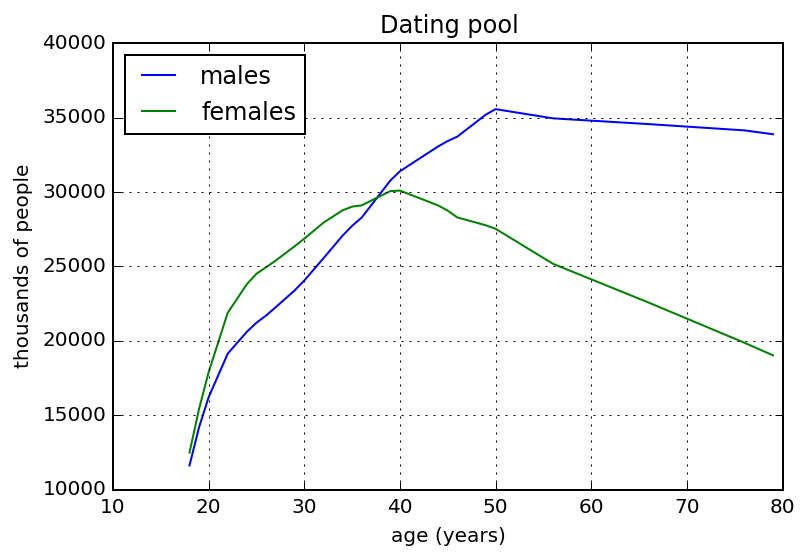So, to comment on the comic's conclusion: indeed, the dating pool grows for men and women until ages 50 and 40, respectively. However, this assumes that people all share the standard creepiness function.

What if instead we assumed a more "normal" rule for dating bounds, say +- 5 years. We can easily plot this curve to see what it looks like.

In :
def dating_pool2(age, dataset):
age_bounds = (age - 5, age + 5)
return singles_between_bounds(age_bounds, dataset)

ages = arange(18, 80)
pool = [dating_pool2(age, data_females) for age in ages]
plot(ages, pool, label='males')
pool = [dating_pool2(age, data_males) for age in ages]
plot(ages, pool, label='females')
title("Dating pool")
xlabel("age (years)")
ylabel("thousands of people")
legend(loc='upper right')
grid(True)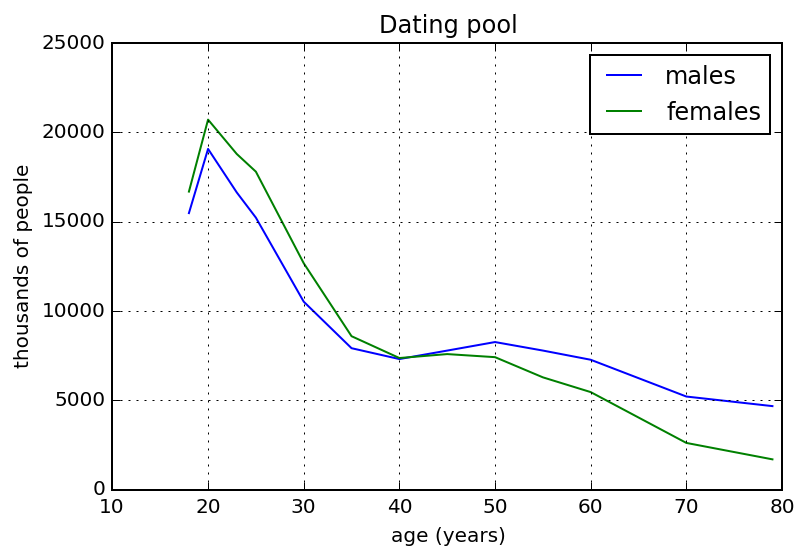So here we have it: if you're not dating at the edge of the bell curve in terms of age (unlike the guy in the webcomic's last panel), your dating pool does go down with age, even though there is a slight rebound between the ages of 40 and 50.

I hope you have enjoyed this little analysis as much as I have writing it. Feel free to comment if you spot some inaccuracies or have some remarks.

This post was entirely written using the IPython notebook. Its content is BSD-licensed. You can see a static view or download this notebook with the help of nbviewer at 20150131_XKCDDatingPools.ipynb.